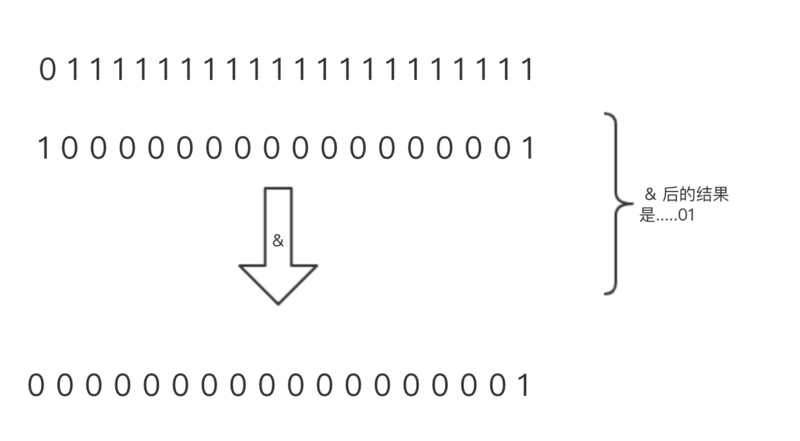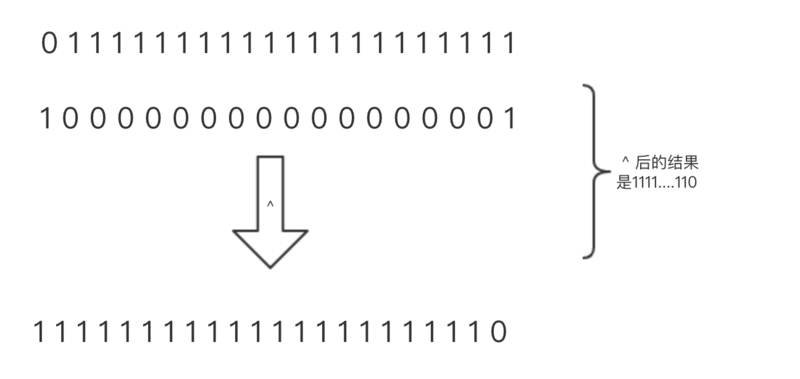# 一道看似简单的面试题

• 时间:# 前言

``````function exponentiation(\$number)
{
if (\$number < 0) {
return false;
}

if ((\$number & (\$number - 1)) == 0) {
return true;
} else {
return false;
}
}

exponentiation (2);
exponentiation (8);
exponentiation (16);
exponentiation (32);
exponentiation (128);
``````

# 运算

## PHP位运算符 (部分)

http://php.net/manual/zh/language.operators.bitwise.php

&按位与运算符
^按位异或运算符

## 按位与``````2 & 3 -> 0010 & 0011 -> 0010 = 2
10 & 7 -> 1010 & 0111 -> 0010 = 2
32 & 70 -> 0100000 & 1000110 -> 0000000 = 0
``````

## 按位异或``````2 ^ 3 -> 0010 ^ 0011 -> 0001 = 1
10 ^ 7 -> 1010 ^ 0111 -> 1101 = 13
32 ^ 70 -> 0100000 ^ 1000110 -> 1100110 = 102
``````

# 进制

n公式结果
122
22x24
32x2x28
42x2x2x216

n公式结果
12000010
22x2000100
32x2x2001000
42x2x2x2010000

``````16 & (16 - 1) =
010000 & (001111) = 0
``````

# 补位

``````01
011
<------>
001
011
``````

# 正负

``````10000000000
01111111111
``````

# 补充

``````function power(\$number){
if (\$number < 0) {
return false;
}

if ((\$number & (\$number - 1)) == 0) {

// 数学不好的,就看下面的方法
// \$number = decbin(\$number);
// return (mb_strlen(\$number)-1);
// 数学可以的就看下面的方法
return floor(log(\$number,2));
} else {
return false;
}
}
``````
1. 判断是否是2的n次方
2. 如果是则将十进制数字转为二进制
3. 计算总长度-1获取到是2的几次方,按照0的个数来计算